Anzeige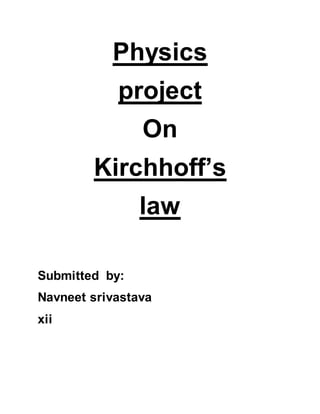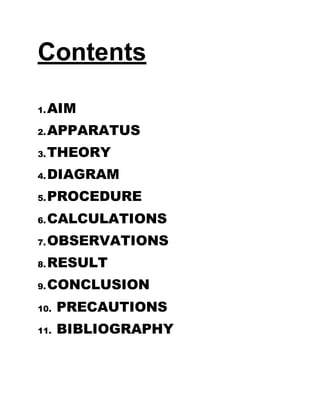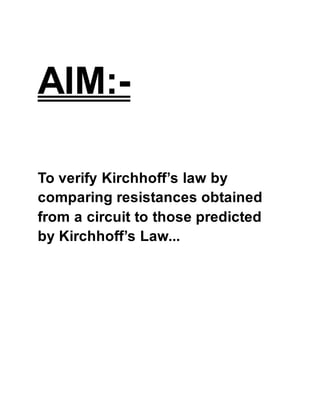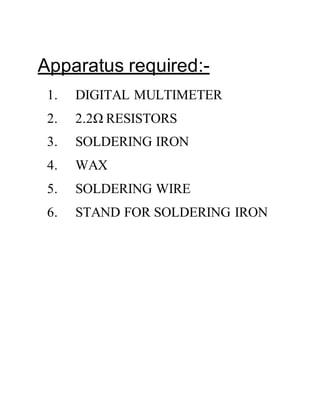Anzeige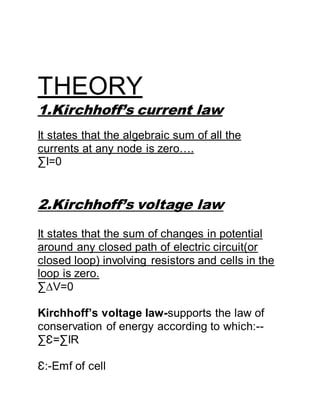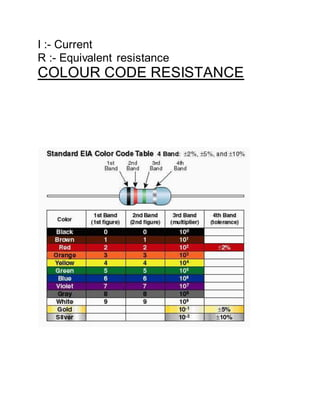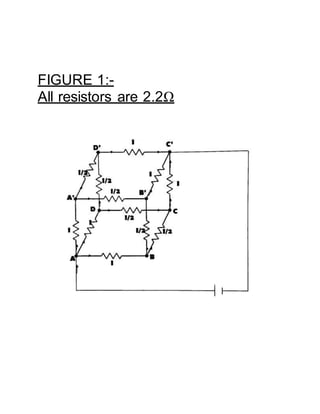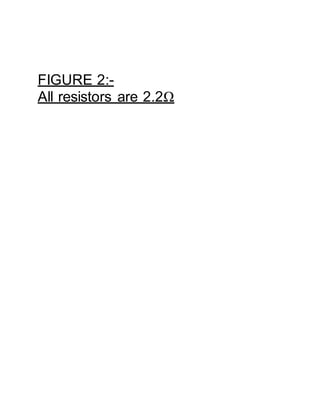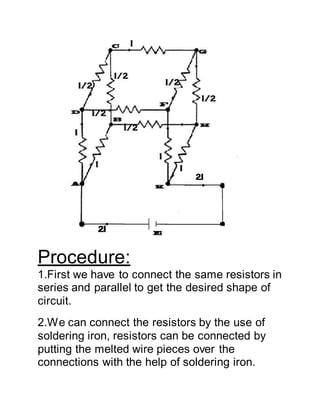Anzeige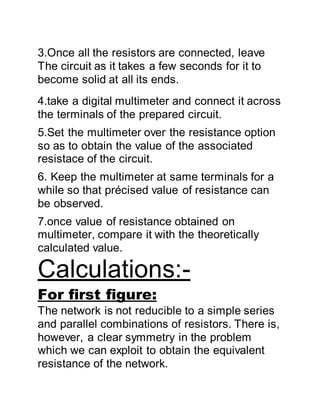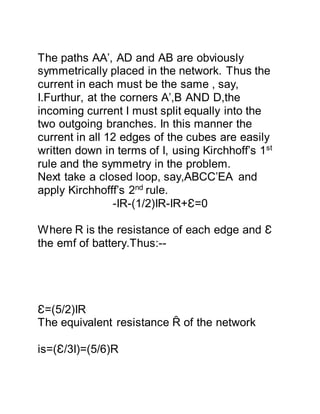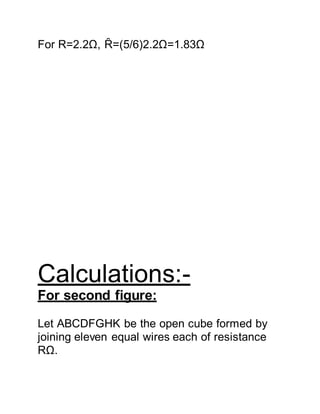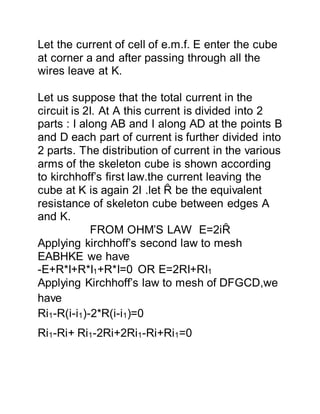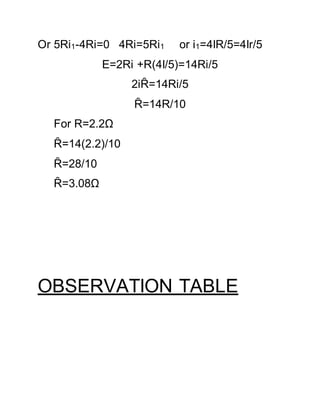Anzeige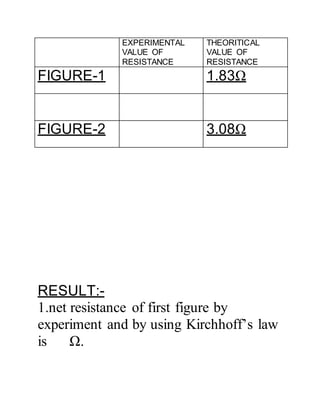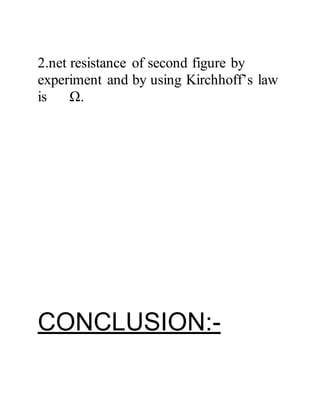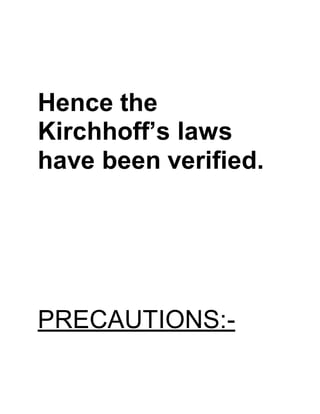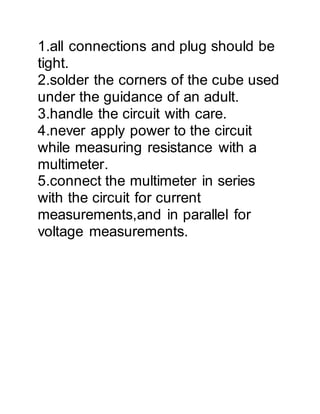Nächste SlideShare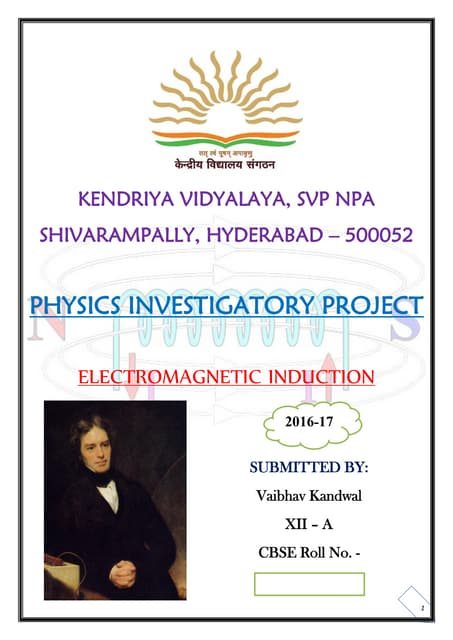CBSE Class XII Physics Investigatory Project
1 von 18
Anzeige

### 350737461-Physics-class-12-project.docx

1. Physics project On Kirchhoff’s law Submitted by: Navneet srivastava xii
2. Contents 1.AIM 2.APPARATUS 3.THEORY 4.DIAGRAM 5.PROCEDURE 6.CALCULATIONS 7.OBSERVATIONS 8.RESULT 9.CONCLUSION 10. PRECAUTIONS 11. BIBLIOGRAPHY
3. AIM:- To verify Kirchhoff’s law by comparing resistances obtained from a circuit to those predicted by Kirchhoff’s Law...
4. Apparatus required:- 1. DIGITAL MULTIMETER 2. 2.2Ω RESISTORS 3. SOLDERING IRON 4. WAX 5. SOLDERING WIRE 6. STAND FOR SOLDERING IRON
5. THEORY 1.Kirchhoff’s current law It states that the algebraic sum of all the currents at any node is zero…. ∑I=0 2.Kirchhoff’s voltage law It states that the sum of changes in potential around any closed path of electric circuit(or closed loop) involving resistors and cells in the loop is zero. ∑∆V=0 Kirchhoff’s voltage law-supports the law of conservation of energy according to which:-- ∑Ɛ=∑IR Ɛ:-Emf of cell
6. I :- Current R :- Equivalent resistance COLOUR CODE RESISTANCE
7. FIGURE 1:- All resistors are 2.2Ω
8. FIGURE 2:- All resistors are 2.2Ω
9. Procedure: 1.First we have to connect the same resistors in series and parallel to get the desired shape of circuit. 2.We can connect the resistors by the use of soldering iron, resistors can be connected by putting the melted wire pieces over the connections with the help of soldering iron.
10. 3.Once all the resistors are connected, leave The circuit as it takes a few seconds for it to become solid at all its ends. 4.take a digital multimeter and connect it across the terminals of the prepared circuit. 5.Set the multimeter over the resistance option so as to obtain the value of the associated resistace of the circuit. 6. Keep the multimeter at same terminals for a while so that précised value of resistance can be observed. 7.once value of resistance obtained on multimeter, compare it with the theoretically calculated value. Calculations:- For first figure: The network is not reducible to a simple series and parallel combinations of resistors. There is, however, a clear symmetry in the problem which we can exploit to obtain the equivalent resistance of the network.
11. The paths AA’, AD and AB are obviously symmetrically placed in the network. Thus the current in each must be the same , say, I.Furthur, at the corners A’,B AND D,the incoming current I must split equally into the two outgoing branches. In this manner the current in all 12 edges of the cubes are easily written down in terms of I, using Kirchhoff’s 1st rule and the symmetry in the problem. Next take a closed loop, say,ABCC’EA and apply Kirchhofff’s 2nd rule. -IR-(1/2)IR-IR+Ɛ=0 Where R is the resistance of each edge and Ɛ the emf of battery.Thus:-- Ɛ=(5/2)IR The equivalent resistance Ȓ of the network is=(Ɛ/3I)=(5/6)R
12. For R=2.2Ω, Ȓ=(5/6)2.2Ω=1.83Ω Calculations:- For second figure: Let ABCDFGHK be the open cube formed by joining eleven equal wires each of resistance RΩ.
13. Let the current of cell of e.m.f. E enter the cube at corner a and after passing through all the wires leave at K. Let us suppose that the total current in the circuit is 2I. At A this current is divided into 2 parts : I along AB and I along AD at the points B and D each part of current is further divided into 2 parts. The distribution of current in the various arms of the skeleton cube is shown according to kirchhoff’s first law.the current leaving the cube at K is again 2I .let Ȓ be the equivalent resistance of skeleton cube between edges A and K. FROM OHM’S LAW E=2iȒ Applying kirchhoff’s second law to mesh EABHKE we have -E+R*I+R*I1+R*I=0 OR E=2RI+RI1 Applying Kirchhoff’s law to mesh of DFGCD,we have Ri1-R(i-i1)-2*R(i-i1)=0 Ri1-Ri+ Ri1-2Ri+2Ri1-Ri+Ri1=0
14. Or 5Ri1-4Ri=0 4Ri=5Ri1 or i1=4IR/5=4Ir/5 E=2Ri +R(4I/5)=14Ri/5 2iȒ=14Ri/5 Ȓ=14R/10 For R=2.2Ω Ȓ=14(2.2)/10 Ȓ=28/10 Ȓ=3.08Ω OBSERVATION TABLE
15. EXPERIMENTAL VALUE OF RESISTANCE THEORITICAL VALUE OF RESISTANCE FIGURE-1 1.83Ω FIGURE-2 3.08Ω RESULT:- 1.net resistance of first figure by experiment and by using Kirchhoff’s law is Ω.
16. 2.net resistance of second figure by experiment and by using Kirchhoff’s law is Ω. CONCLUSION:-
17. Hence the Kirchhoff’s laws have been verified. PRECAUTIONS:-
18. 1.all connections and plug should be tight. 2.solder the corners of the cube used under the guidance of an adult. 3.handle the circuit with care. 4.never apply power to the circuit while measuring resistance with a multimeter. 5.connect the multimeter in series with the circuit for current measurements,and in parallel for voltage measurements.
Anzeige